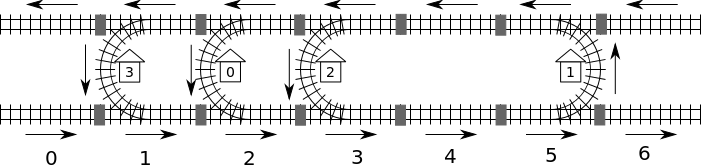# #24. 【IOI2014】Rail### 任务

• findLocation(n, first, location, stype)
• n: 车站的数目
• first: 车站 $0$ 所在区段的编号。
• location: 大小为 $n$ 的数组； 你需要将车站 $i$ 所在的区段编号存放到 location[i] 中。
• stype: 大小为 $n$ 的数组；你需要将车站 $i$ 的类型存放到 stype[i] 中：其中 1 表示 C 类型，而 2 则表示 D 类型。

• getDistance(i, j) 将返回车站 $i$ 到车站 $j$ 的距离。如果调用 getDistance(i, i) 将返回$0$。如果 $i$ 或 $j$ 在 $0 \leq i, j \leq n - 1$ 范围之外，getDistance(i, j) 将返回 $-1$。

### 子任务

18$1 \leq n \leq 100$无限制除车站 $0$ 外，其他车站均在 D 类型区段中。
222$1 \leq n \leq 100$无限制所有在车站 $0$ 东侧的车站均在 D 类型区段中，

326$1 \leq n \leq 5000$$n(n - 1) / 2无其他限定 4441 \leq n \leq 5000$$3 (n - 1)$无其他限定

### 实现细节

void findLocation(int n, int first, int location[], int stype[]);

int getDistance(int i, int j);

### 评测方式

1. 第 $1$ 行: 子任务编号
2. 第 $2$ 行: $n$
3. 第 $3+i$ 行，$(0 \leq i \leq n - 1)$：stype[i]（1表示C类型，2表示D类型）, location[i]。